The OpenFOAM Foundation
geometricOneField Class Reference

A class representing the concept of a GeometricField of 1 used to avoid unnecessary manipulations for objects which are known to be one at compile-time. More...

Inheritance diagram for geometricOneField: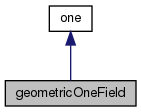[legend]
Collaboration diagram for geometricOneField:[legend]

## Public Types

typedef oneField Internal

typedef oneField Patch

typedef oneFieldField Boundary

typedef one cmptTypePublic Types inherited from one
typedef one value_type

## Public Member Functions

geometricOneField ()
Construct null. More...

const dimensionSetdimensions () const

one operator[] (const label) const

oneField field () const

oneField oldTime () const

Internal operator() () const

Internal v () const

Internal::FieldType primitiveField () const

Boundary boundaryField () constPublic Member Functions inherited from one
one ()
Construct null. More...

operator label () const
Return 1 for label. More...

operator float () const
Return 1 for float. More...

operator double () const
Return 1 for double. More...

operator long double () const
Return 1 for double. More...

## Detailed Description

A class representing the concept of a GeometricField of 1 used to avoid unnecessary manipulations for objects which are known to be one at compile-time.

Used for example as the density argument to a function written for compressible to be used for incompressible flow.

Definition at line 52 of file geometricOneField.H.

## ◆ Internal

 typedef oneField Internal

Definition at line 61 of file geometricOneField.H.

## ◆ Patch

 typedef oneField Patch

Definition at line 62 of file geometricOneField.H.

## ◆ Boundary

 typedef oneFieldField Boundary

Definition at line 63 of file geometricOneField.H.

## ◆ cmptType

 typedef one cmptType

Definition at line 64 of file geometricOneField.H.

## ◆ geometricOneField()

 geometricOneField ( )
inline

Construct null.

Definition at line 69 of file geometricOneField.H.

Here is the call graph for this function: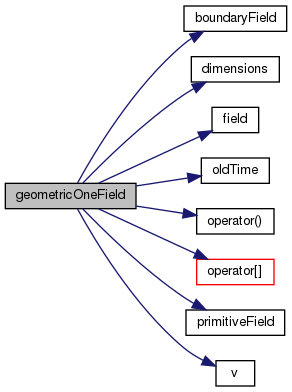## ◆ dimensions()

 const Foam::dimensionSet & dimensions ( ) const
inline

Definition at line 30 of file geometricOneFieldI.H.

References Foam::dimless.

Referenced by geometricOneField::geometricOneField().

Here is the caller graph for this function: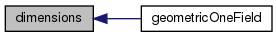## ◆ operator[]()

 Foam::one operator[] ( const label ) const
inline

Definition at line 36 of file geometricOneFieldI.H.

References one::one().

Referenced by geometricOneField::geometricOneField().

Here is the call graph for this function: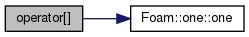Here is the caller graph for this function: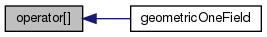## ◆ field()

 Foam::oneField field ( ) const
inline

Definition at line 42 of file geometricOneFieldI.H.

Referenced by geometricOneField::geometricOneField().

Here is the caller graph for this function: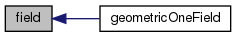## ◆ oldTime()

 Foam::oneField oldTime ( ) const
inline

Definition at line 48 of file geometricOneFieldI.H.

Referenced by geometricOneField::geometricOneField().

Here is the caller graph for this function: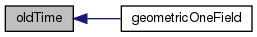## ◆ operator()()

 Foam::geometricOneField::Internal operator() ( ) const
inline

Definition at line 55 of file geometricOneFieldI.H.

Referenced by geometricOneField::geometricOneField().

Here is the caller graph for this function: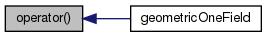## ◆ v()

 Foam::geometricOneField::Internal v ( ) const
inline

Definition at line 62 of file geometricOneFieldI.H.

Referenced by geometricOneField::geometricOneField().

Here is the caller graph for this function: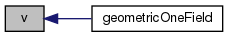## ◆ primitiveField()

 Foam::geometricOneField::Internal::FieldType primitiveField ( ) const
inline

Definition at line 69 of file geometricOneFieldI.H.

Referenced by geometricOneField::geometricOneField().

Here is the caller graph for this function: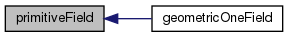## ◆ boundaryField()

 Foam::geometricOneField::Boundary boundaryField ( ) const
inline

Definition at line 76 of file geometricOneFieldI.H.

Referenced by geometricOneField::geometricOneField().

Here is the caller graph for this function: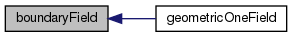The documentation for this class was generated from the following files: